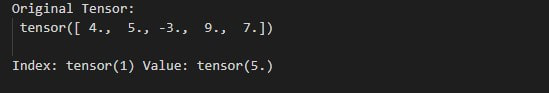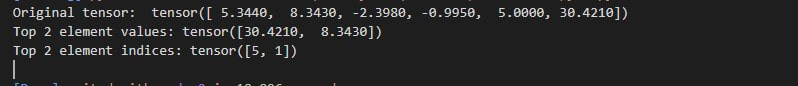Open in App
Not now

# How to find the k-th and the top “k” elements of a tensor in PyTorch?

• Last Updated : 21 Feb, 2022

In this article, we are going to see how to find the kth and the top ‘k’ elements of a tensor.

So we can find the kth element of the tensor by using torch.kthvalue() and we can find the top ‘k’ elements of a tensor by using torch.topk() methods.

• torch.kthvalue() function: First this function sorts the tensor in ascending order and then returns the kth element of the sorted tensor and the index of the kth element from the original tensor.

Syntax: torch.kthvalue(input_tensor, k, dim=None, keepdim=False, out=None)

Parameters:

• Input_tensor: tensor.
• k: k is integer and it’s for k-th smallest element of tensor.
• dim: dim is for dimension to find the k-th value along of tensor.
• keepdim (bool): keepdim is for whether the output tensor has dim retained or not.

Return: This method returns a tuple (values, indices) of the k-th element of tensor.

• torch.topk() function: This function helps us to find the top ‘k’ elements of a given tensor. it will return top ‘k’ elements of the tensor and it will also return indexes of top ‘k’ elements in the original tensor.

Syntax: torch.topk(input_tensor, k, dim=None, largest=True, sorted=True, out=None)

Parameters:

• input_tensor: tensor.
• k: k is integer value and it’s for the k in top-k.
• dim: the dim is for the dimension to sort along of tensor.
• largest: this is used to controls whether return largest or smallest elements of tensor.
• sorted: it controls whether to return the elements in sorted order.

Return: this function is returns the ‘k’ largest elements of tensor along a given dimension.

Example 1: The following program is to find the k-th element of a tensor.

## Python3

 `# import torch library``import` `torch`` ` `# define a tensor``tens ``=` `torch.Tensor([``4``, ``5``, ``-``3``, ``9``, ``7``])``print``(``"Original Tensor:\n"``, tens)`` ` `# find 3 largest element from the tensor``value, index ``=` `torch.kthvalue(tens, ``3``)`` ` `# print value along with index``print``(``"\nIndex:"``, index, ``"Value:"``, value)`

Output:Example 2: The following program is to find the top k elements of tensor

## Python3

 `# import torch library``import` `torch`` ` `# define tensor``tens ``=` `torch.Tensor([``5.344``, ``8.343``, ``-``2.398``, ``-``0.995``, ``5``, ``30.421``])``print``(``"Original tensor: "``, tens)`` ` `# find top 2 elements``values, indexes ``=` `torch.topk(tens, ``2``)`` ` `# print top 2 elements``print``(``"Top 2 element values:"``, values)`` ` ` ` `# print index of top 2 elements``print``(``"Top 2 element indices:"``, indexes)`

Output:My Personal Notes arrow_drop_up